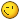Name E-mail Your e-mail address will never be displayed on the site. Subject Message

## Topic History of : Depreciation Rate formula

Max. showing the last 6 posts - (Last post first)
11 years 3 months ago #397

Erik Rudd, ITIL-F, PMPAlexander,

I appreciate that you learned depreciation long ago and learned to calculate as a factor. That's not the point I was trying to make. The point is one of communicating the concept to those who may not already be familiar with the depreciation formulas in the most easily grasped concept. Of course a person familiar with factoring will use a whole to represent 100% but it's easier to explain to someone that you'd use 100% of \$120k than it is explaining how you'd use 1 to represent 100% of a \$120k.

Please don't misunderstand...I'm not advocating that you change what you use for calculating depreciation.
11 years 3 months ago #396

ALEXANDER CHISTOVErik,

Never mind. If it is more convenient for you to understand the formula with % designator and to calculate in percent - do what you like and forget what I say.

For me 'rate' is the same thing as 'factor' or 'coefficient' and it must not be necessarily in percent, it could be just a figure which I use for further calculations, when applying this rate to something.

Just forget it. I learned depreciation stuff long ago and it happened so that I got used to use 1 in the formula. Never had any trouble.

Thanks for your attention in any case.
11 years 3 months ago #395

Erik Rudd, ITIL-F, PMPAlexander,

Take Cornelius' example of an item costing \$120,000.00 with a useful life of 5 years and plug it into the formula as Cornelius has it written and then plug it into the formula as you'd like to see it written.

I understand from Cornelius' example to plug in 120,000 as 100% of the initial cost...I would have no idea what to plug into your example, 1 of what??.
11 years 3 months ago #394

ALEXANDER CHISTOVCornelius,
I did not tell you that you are wrong. In my first message I stated that it is technically correct, but is confusing visually.
I am not telling that in your example 40% is not correct, I am telling that for further calculation it is easier to use 0.4 (which is the same thing) than anything with '%'
If I use a simple calculator for a fast estimation of depreciation value, I don't even know how could I input this '40%' into the device, while '0.4' makes it a lot quicker to use in any calculation.
So, we are talking the same thing, but we see it from different angles. Try to understand me. When I need to calculate amount, I care about money and to get that value I would use figure '0.4' in calculations.
So, 100% or 1 (just figure, not percent!) are both correct for the formula, but for my personal taste '1' is easier to understand.

p.s. Thanks a lot for the useful link!
11 years 3 months ago #393

Cornelius FichtnerAlexander,

If I'm not totally mistaken, then you are referring to the formula for the double declining balance on page 6 at the top. The formula is correct.

If you have an asset that costs \$120,000 and has a useful life of 5 years, then the depreciation rate for the double declining balance is calculated as follows:

Depreciation Rate = 2 * (100% / Useful Life)
Depreciation Rate = 2 * (100% / 5)
Depreciation Rate = 2 * (20%)
Depreciation Rate = 40%

And yes... your yearly depreciation is really 40% of the book value at the beginning of the year. It sounds high but makes sense when you think about it logically: That way you depreciate a higher value in the early life of your asset, which is usually where the depreciation is highest anyway. (Like when you drive your new car out of the dealer lot you are "losing" about 30% value at that very moment...So using 100 in the formula is correct. 1 would be wrong.

In any case, take a look at the excellent description of this on Wikipedia. The tables they show make it really come alive: en.wikipedia.org/wiki/Depreciation
11 years 3 months ago #392

ALEXANDER CHISTOVHi,
I've spotted a confusing point in the "Formula Study Guide"(TM). It says: Depreciation Rate = 2 * (100% / Useful Life), which is technically correct, but usage of '100%' notation instead of just figure '1' could potentially lead to miscalculation. Not everybody would recall it naturally that 100% = 1 and somebody might do a wrong math deviding 100% literally like 100.
Can you imagine what their Depriciation Expense would be afterwards?

OSP INTERNATIONAL LLC
OSP INTERNATIONAL LLC
Training for Project Management Professional (PMP)®, PMI Agile Certified Practitioner (PMI-ACP)®, and Certified Associate in Project Management (CAPM)®